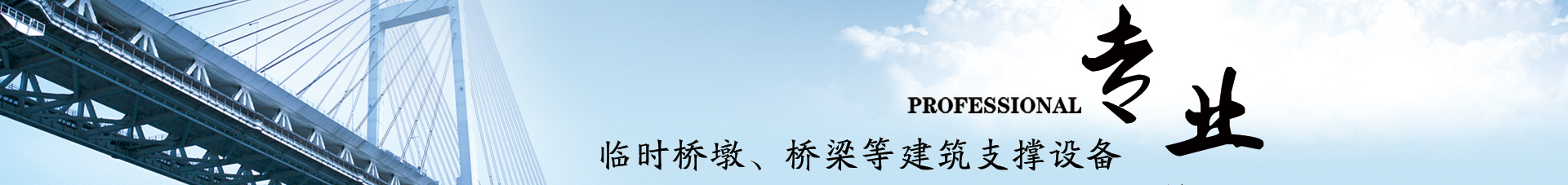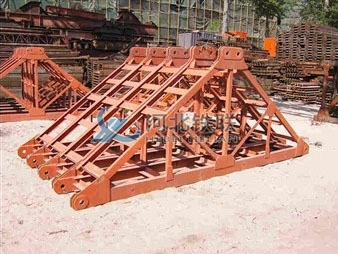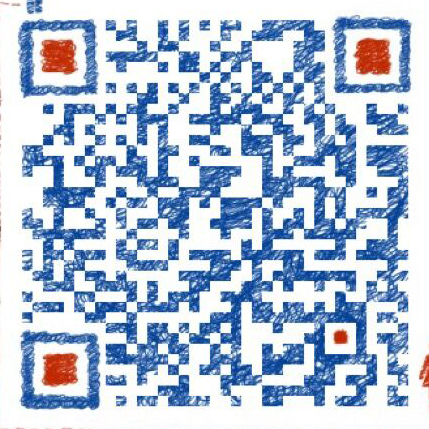# 如果贝雷片作为纵梁需要怎么计算

### 发布日期：2018-05-08 作者： 点击：3、施工人员、施工料具运输堆放荷载  ①计算模板及直接支承模板小棱时，均布荷载可取2.5kPa。 ②计算直接支承小棱的梁或拱架时，均布荷载可取1.5 kPa。 ③计算支架立柱及支承拱架的其它结构件时，均布荷载可取1.0kPa。  4、倾倒混凝土时产生的冲击荷载按2.0kN/㎡。 5、振捣混凝土产生的荷载按2.0kN/㎡。  6、其它可能产生的荷载，如雪荷载、冬季保温设施荷载等，基本不产生。  7、新浇混凝土对模板侧面压力，泵送时：Pm=4.6v1/4=4.6×21/4=5.5 kN/㎡。  8、倾倒混凝土时对侧面模板产生的水平荷载按2.0kN/㎡。 9、振捣混凝土产生时对侧面模板产生的压力按4.0kN/㎡。单跨现浇箱梁重：（522.2+1.08）m3*2600/80×23.5=399655.1Kg ⑵扩大基础自重： 32.78m3*2600=85228Kg  ⑶模板、支架、其他重：（0.75+1.5+2）*10*23.5=99875Kg ⑷贝雷片重：287*16*4=32144Kg 两侧扩大基础承载力：450kpa×11×1.5=7425KN > （399655.1+99875+44136+42614）×10/（4×1000）=1465.7KN。 满足要求。  此荷载为简支荷载，因此钢管桩基础承载力为：（399655.1+99875+44136）×10/（2×1000）=2718.3KN贝雷架材料允许弯曲应力  [σ]=273 Mpa 贝雷架材料允许剪应力    [σ]=208 Mpa  贝雷片承受荷载主要是箱梁梁体、支架、自重等荷载。为均布荷载 底板下三个贝雷纵梁  q=（3.875×26+5.55×（0.75+1.5+2.0+2.0）+5.74）/3=47.06KN/m   最大弯矩可能在M①中、MB支 M①中=0.07ql2=474.356KN.m MB支=0.125ql2=847.065 KN.m σ max  =Mmax/w=118.35Mpa<[﹠]=273Mpa.安全系数2.31.满足要求QB左=0.625ql=352.943＜【490.5】KN 绕度计算:  工字钢容许绕度[f]为（1/200~1/1000）L  这里取L/800=1.5cm fmax=0.521ql4/(100EI)=4.83mm < [f]=1.5cm  满足要求。 外侧2个贝雷纵梁  q=（(0.7088×26+2.266×(0.75+1.5+4)+3.8267)/2=18.209KN/m   最大弯矩可能在M①中、MB支 M①中=0.07ql2=183.547KN.m Mb支=0.125ql2=327.762 KN.m σ max  =Mmax/w=45.795Mpa<[﹠]=273Mpa.安全系数5.96.满足要求。  剪力最大可能在QA、QB左  QA=0.375ql=81.941KN＜【490.5】KN QB左=0.625ql=136.568＜【490.5】KN 绕度计算:  工字钢容许绕度[f]为（1/200~1/1000）L  这里取L/800=1.5cm f=0.521ql4/(100EI)=1.9*10-6mm < [f]=1.5cm  满足要求。 支架计算 底板支架  支架承受荷载主要集中的箱梁底板。  P=3.87526/5.55+0.75+1.5+2+2=24.403KN/m2  设计支架间距为：横距0.9m，纵距0.6m。横杆步距1.2m。 立杆伸出顶层水平杆中心至模板支撑点长度，取40cm.每根立杆承重：N=24.403 KN/m2*0.9m*0.6m=13.177N 钢管用￠48×3mm，A为截面积=424mm2 钢管回转半径i=2√R²+r² =15.9mm  长细比为 入=（1200+2*400）/15.9=125.8 查表的￠=0.417  支柱的收压应力为：σ=N/（￠A）=13177/(0.417*424)=74.527Mpa < 215Mpa.满足要求。 翼板支架  P=0.7088×26/2.25+0.75+1.5+2+2=14.44KN/m2  设计支架间距为：横距0.9m，纵距0.6m。横杆步距1.2m。 立杆伸出顶层水平杆中心至模板支撑点长度，取40cm. 每根立杆承重：N=24.403 KN/m2*0.9m*0.6m=7.798N 钢管用￠48×3mm，A为截面积=424mm2 钢管回转半径i=2√R²+r² =15.9mm  长细比为 入=（1200+2*400）/15.9=125.8 查表的￠=0.417  支柱的收压应力为：σ=N/（￠A）=7798/(0.417*424)=44.104Mpa < 215Mpa.满足要求。  细部结构检算  1、底模下的10×10cm方木棱,最不利荷载在腹板底  跨度 l=0.9m(间距0.35m)  均布荷载 q=0.35×1.4×26+（0.75+1.5+2.0+2.0）×0.35=14.93kN/m  Mmax = ql2/8=(8.22×103×0.92)/8=1511.4N·m σ max = Mmax/W=832.28/ (0.13/6) =9.07Mpa＜ Mpa安全系数为11/9.07=1.21，满足要求。  fmax=5ql4/384EI=(5×8.22×103×0.94)/[384×9×109× (0.14/12)] =1.7mm＜3.6 mm=l/250 ， 满足要求。2、10×10cm方木棱下的15×15cm方木纵梁  跨度 l=0.9m（纵距0.6m）  均布荷载 q=0.6×1.4×26+（0.75+1.5+2.0+2.0）×0.6=25.6kN/m  Mmax = ql2/8=(18.5×103×1.22)/8=2591N·m σ max = Mmax/W=3330/ (0.153/6) =4.61Mpa＜ Mpa，安全系数为11/4.61=2.39，满足要求。  fmax=5ql4/384EI=(5×18.5×103×1.24)/[384×9×109× (0.154/12)] =0.58mm＜4.8 mm=l/250 ， 满足要求。3、木内模跨间4×6cm支承底模方木棱  跨度 l=0.6m

### 相关标签：贝雷梁

#### 相关新闻：